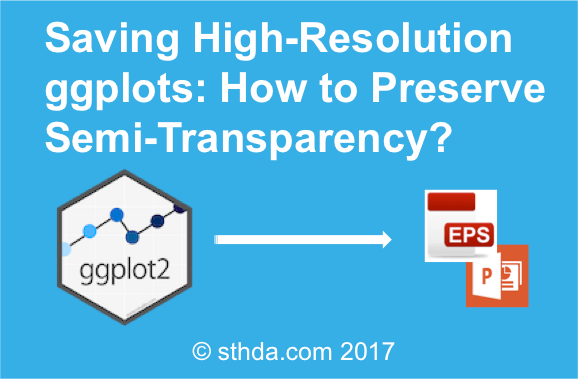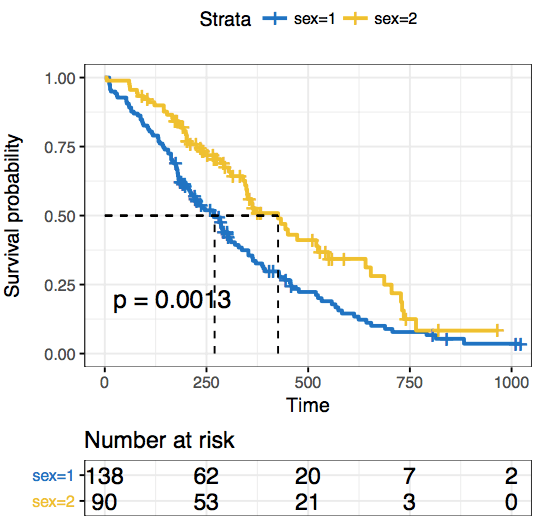Want to share your content on R-bloggers? click here if you have a blog, or here if you don't.

This article describes solutions for preserving semi-transparency when saving a ggplot2-based graphs into a high quality postscript (.eps) file format.Contents:

## Create a ggplot with semi-transparent color

To illustrate this, we start by creating ggplot2-based survival curves using the function ggsurvplot() in the survminer package. The ggsurvplot() function creates survival curves with the 95% confidence bands in a semi-transparent color.

First install (if needed) survminer as follow:

`install.packages("survminer")`

Then type, this:

```# Fit survival curves
require("survival")
fit<- survfit(Surv(time, status) ~ sex, data = lung)

# Visualize
library("survminer")
p <- ggsurvplot(fit, data = lung,
surv.median.line = "hv", # Add medians survival
pval = TRUE,             # Add p-value and tervals

conf.int = TRUE,        # Add the 95% confidence band
risk.table = TRUE,      # Add risk table
tables.height = 0.2,
tables.theme = theme_cleantable(),
palette = "jco",
ggtheme = theme_bw()
)
print(p)```In the plot above, the confidence band is semi-transparent. It can be saved to a PDF file without loosing the semi-transparent color.

If you try to export the picture as vector file (EPS or SVG, …), the 95% confidence interval will disappear and the saved plot looks as follow:The problem is that EPS in R does not support transparency.

In the following sections, we’ll describe convenient solutions to save high-quality ggplots by preserving semi-transparency.

## Save ggplots with semi-transparent colors

### Use cairo-based postscript graphics devices

You can use the ggsave() function in [ggplot2] as follow:

```ggsave(filename = "survival-curves.eps",
plot = print(p),
device = cairo_eps)```

Or use this:

```cairo_ps(filename = "survival-curves.eps",
width = 7, height = 7, pointsize = 12,
fallback_resolution = 300)

print(p)

dev.off()```

Note that, the argument fallback_resolution is used to control the resolution in dpi at which semi-transparent areas are rasterized (the rest stays as vector format).

### Export to powerpoint

You can export the plot to Powerpoint using the ReporteRs package. ReporteRs will give you a fully editable vector format with full support for transparency as well.

We previously described how to Create and format PowerPoint documents from R software using the ReporteRs package. We also described how to export an editable ggplot from R software to powerpoint.

Briefly, to export our survival curves from R to powerpoint, the script looks like this

```library('ReporteRs')

# Create a new powerpoint document
doc <- pptx()

# Add a new slide into the ppt document
doc <- addSlide(doc, slide.layout = "Two Content"  )

doc <- addTitle(doc, "Survival Curves: Editable Vector Graphics" )

# Print the survival curves in the powerpoint
doc <- addPlot(doc, function() print(p, newpage = FALSE),
vector.graphic = TRUE  # Make it editable
)

# write the document to a file
writeDoc(doc, file = "editable-survival-curves.pptx")```

The output looks like this:Edit the plot in powerpoint. See the video below: Editing ggplots Exported with ReporteRs into PWPT